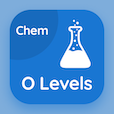Engineering Online Courses

Basic Electronics MCQs

Ece Viva Questions and Answers Multiple Choice Questions (MCQ), Basic Electronics quiz answers PDF to learn basic electronics online course for basic electronics classes. Transconductance Multiple Choice Questions and Answers (MCQs), Basic Electronics quiz questions for online associate degree in engineering. "Ece Viva Questions and Answers" PDF Book: electronics and communication multiple choice questions with answers, pn junction lab viva questions for competitive exams, electronics engineering interview questions, basic electronics questions and answers for interview test prep for employment assessment test.

"If output current of circuit is 12A and input voltage is 3V than transconductance of circuit will be" MCQ PDF: ece viva questions and answers with choices 15v, 15a, 4a, and 4 for online associate degree in engineering. Learn ece viva questions and answers quiz questions for merit scholarship test and certificate programs to enroll in online classes.

## MCQs on Ece Viva Questions and Answers Quiz

MCQ: If output current of circuit is 12A and input voltage is 3V than transconductance of circuit will be

15V
15A
4A
4

MCQ: A temperature sensitive resistor with a negative temperature coefficient is called

thyristor
SCR
thermometer
thermistor

MCQ: Ratio of output current to the input voltage is called

transresistance
inductance
transconductance
gain ratio

MCQ: A three terminal thyristor that can conduct current in either direction when properly activated is called

Diac
Triac
Biac

MCQ: A phenomena of exactly zero electrical resistance and expulsion of magnetic fields occurring in certain materials when cooled below a characteristic critical temperature is called

inductivity
capacitvity
conductivity
superconductivity

### More Topics from Basic Electronics Course